#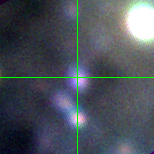M33C-13767 (J013352.39+303920.9)

## Target Notes### Classification: OB-Supergiant

In a crowd with many LGGS stars >3 magnitudes fainter all around it. One pair 2.2 arcseconds away combines to be about 2 magnitudes fainter than the target in all bands. This blend is potentially problematic. I band measures marked "B" because not resolved when it should have according to LGGS. In other bands when the target is confused and not clearly resolved marked "X."

## Plots of Brightness and Color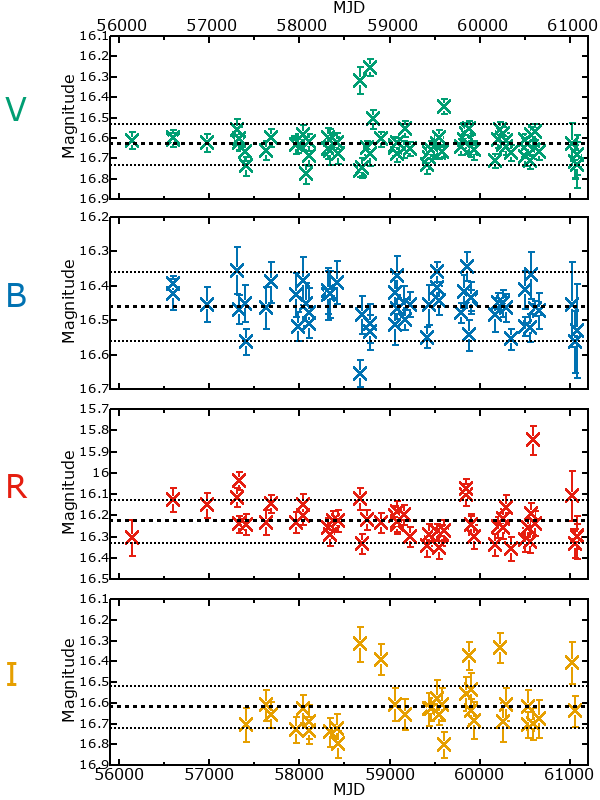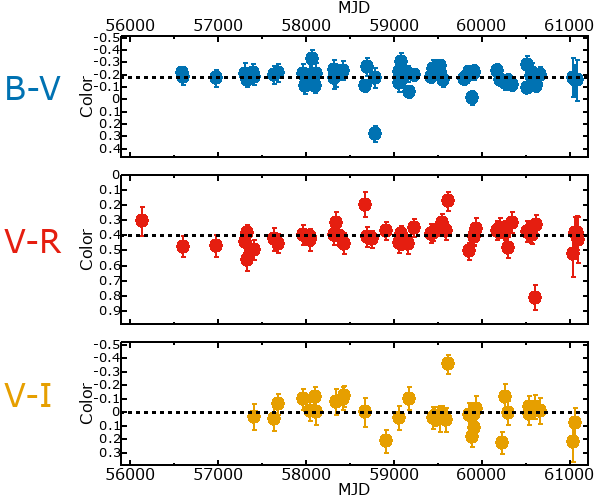## Variability

From the data available in this survey, it is not possible to tell with certainty if this star is variable.

### Correlation factors between bands

If the brightness variation is true variability then there should be a correlation between brightness changes recorded in different filter bands. The following table are the R-squared linear correlation factors calculated for the pairs of bands. The number in parentheses next to the R-squared value is the number of elements used to calculate the correlation factor. The number in square brackets next to the band is the range of magnitudes measured.

V [0.66 mag]B [0.81 mag]R [1.10 mag]
B [0.66 mag]0.02 (30)
R [1.10 mag]0.00 (25)0.33 (17)
I [1.15 mag]0.01 (15)0.02 (9)0.02 (9)

### Magnitude-Magnitude Plots

The plots below are provided to check the values in the table above and also to show any non-linear correlations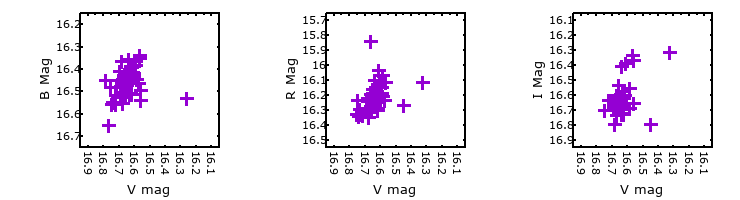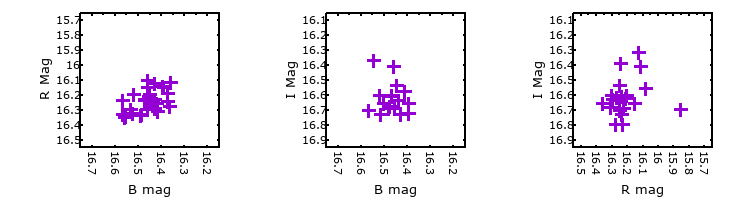### I & R Statistics

Welch & Stetson (1993, AJ, 105, 1813) present a method for varible star detection in CCD images from automated surveys. Data from two bands are compared and two statistics are calculated: R and I.

The I-statistic has an expectation value of zero for stars that are NOT variable. A larger non-zero I-value indicates a higher likelihood that the star is variable. The R statistic indicates how the color of the star changes as the variable grows brighter with R = 1.0 being no change in color.

R and I values have only been calculated if there are more than four (4) matched measurements of brightness in each band.
V
(I|R)
B
(I|R)
R
(I|R)
B
(I|R)
2.49 | 0.69
R
(I|R)
1.40 | 0.857.67 | 0.35
I
(I|R)
-1.81 | 2.00-0.51 | 1.09-0.82 | 1.10